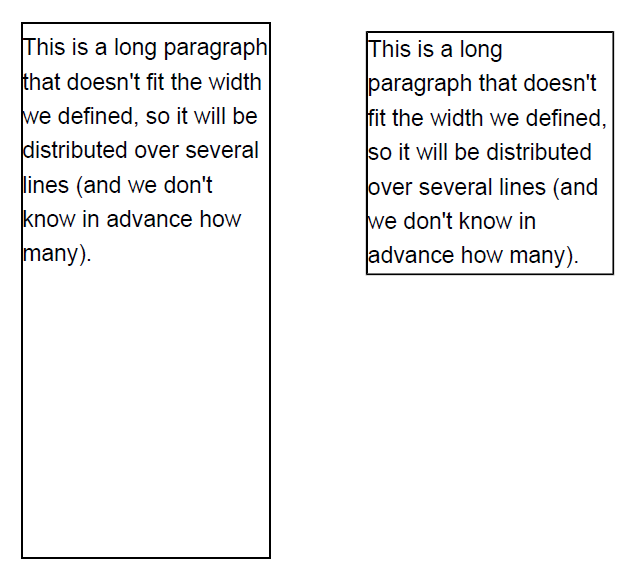How to draw a rectangle around multiline text?

I am trying to draw a rectangle around multi-line text in iText. I use this code to draw the text:

```ColumnText ct = new ColumnText(cb);
Phrase phrase = new Phrase("Some String\nOther string etc...\n test");
ct.setSimpleColumn(myText......);
ct.go();```
I know how to draw a rectangle, but I am not able to draw a rectangle outlining this text.

Posted on StackOverflow on Mar 13, 2012 by user2439522

Let's draw the same paragraph twice. The first time, we'll add a rectangle that does not look like a border for our text. The second time, let's add a rectangle the way we want it to look:Screen shot

The first way is the following:

``````new Canvas(canvas, pdfDoc, new Rectangle(120, 500, 130, 280))
canvas.rectangle(120, 500, 130, 280);
canvas.stroke();
``````

You define the area to add some text using the parameters:

``````x = 120;
y = 500;
width = 130;
height = 280;
``````

This is a rectangle with lower left corner (120, 500), a width of 130 and a height of 280. Hence you draw a rectangle like this:

``````canvas.rectangle(120, 500, 130, 280);
canvas.stroke();
``````

Unfortunately, that rectangle is too big.

And now let's use `setBorder()` method just to envelop the text, no matter how many lines are there:

``````p.setBorder(new SolidBorder(1));
new Canvas(canvas, pdfDoc, new Rectangle(300, 500, 130, 280))
``````

This time you add a paragraph to the `Canvas` with a pre-defined border. It works perfectly!

Click this link if you want to see how to answer this question in iText 5.